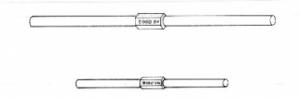# Wb-7 Vibrations of a Rod

## Aim

To demonstrate transverse vibrations in a rod.

## Apparatus

• Rods
• Rubber MalletVibration Rods and Equation.

## Description

DESCRIPTION: The frequencies of transverse vibration of an elastic bar are given by the equation above.

Where E is Young’s Modulus of the material of the rod

ρ is the density of the rod

l is the length of the rod

A) The frequency of transverse vibrations is proportional to the velocity of longitudinal vibrations.

B) The frequency N varies inversely as the square of the length of the bar indicating that the velocity of the transverse vibrations in a bar is dependent on the frequency.

The frequencies of the successive symmetrical modes of vibration of the bar are proportional to  32 72 112 152 ... approx.
the overtones are therefore not harmonics as in the case of in the vibrations of a string. Two bars are available one of length 32 cm diameter 1.584 cm which vibrates at 8000 Hz and the other of length 64 cm diameter 1.644 cm which vibrates at 4000 Hz.